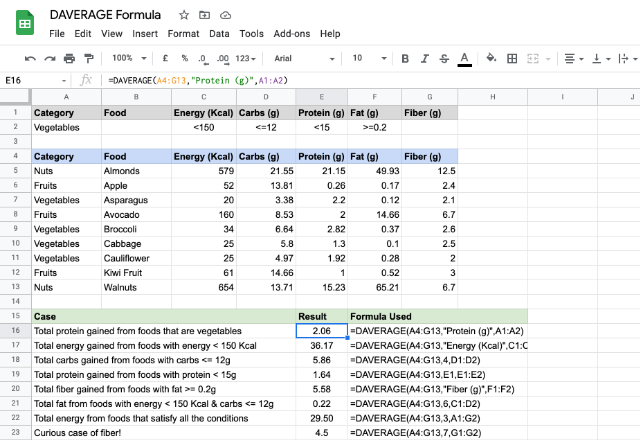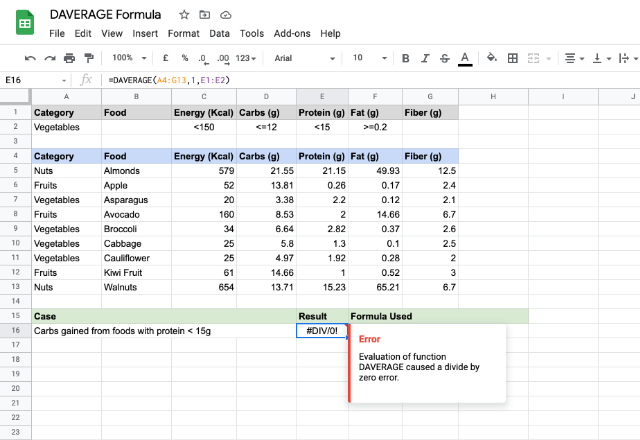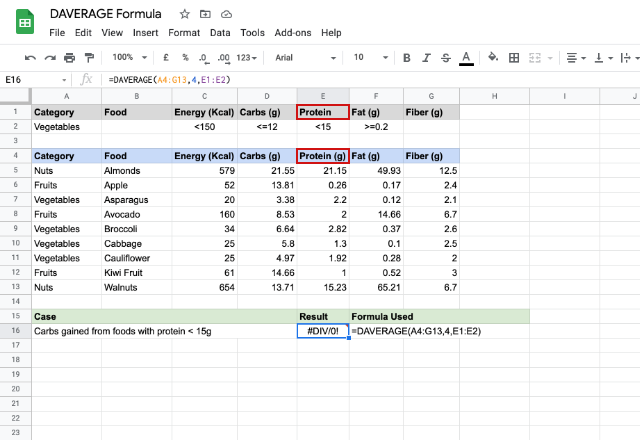# How to use the DAVERAGE formula in Google SheetsThe DAVERAGE formula in Google Sheets is almost similar to that of the AVERAGEformula, but with a distinction. It gives us the average of values available in a table like range, that meet a specified criteria. This is analogous to an SQL database sum query. The ‘D’ in the DAVERAGE stands for ‘Database’, therefore, we can call this a Database AVERAGE formula.

### Syntax

DAVERAGE(database, field, criteria)

• database – is the reference to a structured data range that consists of labels, for each column, in the first row.
• field – indicates the column on which the DAVERAGE formula should average the numeric values. This can be a text or a column index too.
• criteria – this is a reference to a range that consists of criteria that the formula uses to filter the database values before averaging.

### Usage: DAVERAGE Formula

Let us assume that the below list of foods is our secret diet suggested by a nutritionist. We will try our hands with a few examples.We see that the data set is in a labeled tabular form spanning across cells A4 through to G13. And the criteria is keyed in the cells A1 through to G2.

Please note from the cases illustrated above that we can specify one or more criteria to filter the averaging process. For example, the case in row # 7 averages the values in the “Carbs (g)” column, subject to the criteria in the cell D2. Whereas the case in row # 10 averages the values from “Fat (g)” column, subject to the criteria within the cells C2 and D2. The case on row # 11 includes all the criteria from A2 through to G2.

The case in row # 12 is a rather interesting one! We didn’t specify anything in the cell G2, yet, we gave that reference to the DAVERAGE formula. The result is 4.50 because it averaged everything in the “Fiber (g)” column as there are no criteria to filter out the rows.

##### Unwarranted Case

Now, let us talk about a pitfall. Here is the first one, which we might already be aware of. Please consider the first case in the snapshot below.Here, we tried averaging non-numeric values! Just like the AVERAGE formula, DAVERAGE formula averages only numbers. Otherwise, it returns #DIV/0! error.

Here’s the second one in the image below.Seemingly there’s nothing wrong with the formula this time but the result isn’t what we are expecting. The problem lies within the labels though. Apparently, a missing or a mismatched label doesn’t help the DAVERAGE formula, as its working hinges on the field name that the second parameter takes. The labels didn’t match, so it evaluates zero instances of a criteria match, which implies a divide by zero occurrences, hence the error.